# NCERT Solutions for Class 8 Maths Chapter 8 Comparing Quantities Ex 8.3

These NCERT Solutions for Class 8 Maths Chapter 8 Comparing Quantities Ex 8.3 Questions and Answers are prepared by our highly skilled subject experts.

## NCERT Solutions for Class 8 Maths Chapter 8 Comparing Quantities Exercise 8.3

Question 1.
Calculate the amount and compound interest on
(a) ₹ 10,800 for 3 years at 12$$\frac{1}{2}$$% per annum compounded annually.
(b) ₹ 18,000 for 2$$\frac{1}{2}$$ years at 10% per annum compounded annually.
(c) ₹ 62,500 for 1$$\frac{1}{2}$$ years at 8% per annum compounded half yearly.
(d) ₹ 8,000 for 1 year at 9% per annum compounded half yearly.
(You could use the year by year calculation using SI formula to verify)
(e) ₹ 10,000 for 1 year at 8% per annum compounded half-yearly.
Solution:
(a) Here P = ₹ 10800, n = 3 yearsCompound interest = ₹ 15377.34 – ₹ 10,800 = ₹ 4577.34
Amount = ₹ 15377.34
Compound interest = ₹ 4577.34

(b) HereP = ₹ 18,000, n = 2$$\frac{1}{2}$$, R = 10% p.a
For first 2 yearsCI = A – P = 21,780 – 18000 = ₹ 3780
For the last $$\frac{1}{2}$$ year, principal become ₹ 21,780
So, Interest = $$\frac{21780 \times 10 \times 1}{2 \times 100}$$ = ₹ 1089
∴ Total interest = 3780 + 1089 = ₹ 4869(c) Here P = ₹ 62,500, R = $$\frac{8}{2}$$ = 4% per half year
n = $$\frac{3}{2}$$ × 2 = 3 years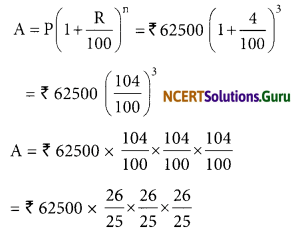A = ₹ 4 × 26 × 26 × 26 = ₹ 70304
Compound interest = Amount – Principal
= ₹ 70304 – ₹ 62500
= ₹ 7804

(d) Here P = ₹ 8000, n = 2 (2 half year),
R = $$\frac{9}{2}$$ % (half yearly)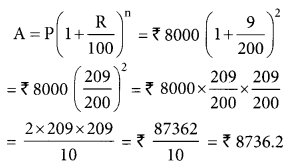Compound interest = ₹ 8736.2 – ₹ 8000 = ₹ 736.20

(e) Here P = ₹ 10,000; n = 2 (2 half year);
R = $$\frac{8}{2}$$ % = 4% (half yearly)Compound Interest = ₹ 10816 – ₹ 10,000 = ₹ 816Question 2.
Kamala borrowed ₹ 26,400 from a Bank to buy a scooter at a rate 15% p.a. compounded yearly. What amount will she pay at the end of 2 years and 4 months to clear the loan?
(Hint: Find A for 2 years with interest is compounded yearly and then find SI on the 2nd year amount for $$\frac{4}{12}$$ years).
Solution:
Here P = ₹ 26,400, R% = 15% p.a, n = 2 yearsSimple interest on ₹ 34914 at 15% p.a for 4 months ($$\frac{1}{3}$$ years)
= ₹ $$\frac{34914 \times 15 \times 1}{100 \times 3}$$
= ₹ 1745.70
Requiredamount = ₹ 34914 + ₹ 1745.70 = ₹ 36,659.70

Question 3.
Fabina borrows ₹ 12,500 at 12% per annum for 3 years at simple interest and Radha borrows the same amount for the same time period at 10% per annum, compounded annually. Who pays more interest and by how much?
Solution:
For Fabina,
P = ₹ 12,500, R% = 12%, T = 3 years
Simple interest = $$\frac{\text { PRT }}{100}$$
= ₹ $$\frac{12500 \times 12 \times 3}{100}$$
= ₹ 4500
P = ₹ 12500, R = 10% p.a. n = 3 yearsCompound interest = Amount – Principal
= ₹ 16,637.50 – ₹ 12500
= ₹ 4137.50
Difference between C.I and S.I = ₹ 4500 – ₹ 4137.50 = ₹ 362.5
Fabina pays more by ₹ 362.50Question 4.
I borrowed ₹ 12,000 from Jamshed at 6% per annum simple interest for 2 years. Had I borrowed this sum at 6% per annum compound interest, what extra amount would I have to pay?
Solution:
Simple interest
Here P = ₹ 12000, R% = 6%, T = 2 years
I = $$\frac{\text { PRT }}{100}$$
= ₹ $$\frac{12000 \times 6 \times 2}{100}$$
= ₹ 1440
Compound interest
Here P = ₹ 12000, R = 6%, n = 2 years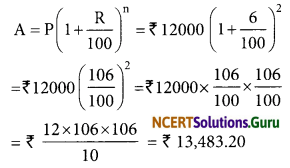C.I. = ₹ 13,483.20 – ₹ 12000 = ₹ 1483.20
∴ Excess amount = ₹ 1483.20 – ₹ 1440 = ₹ 43.20
I would have to pay to him an excess amount of ₹ 43.20.

Question 5.
Vasudevan invested ₹ 60,000 at an interest rate of 12% per annum compounded half yearly. What amount would he get
(i) after 6 months?
(ii) after 1 year?
Solution:
(i) After 6 months
Here, P = ₹ 60,000, R = 12% per annum = $$\frac {1}{2}$$ × 12 = 6%, n = 1 (one half year)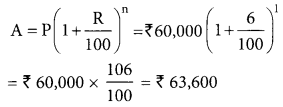He would get ₹ 63,600 after 6 months

(ii) After one year
Here P = ₹ 60,000, R = $$\frac{12}{2}$$ = 6% per half year
n = (1 × 2) = 2 half years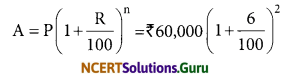Hence, he would get ₹ 67,416 after one yearQuestion 6.
Arif took a loan of ₹ 80,000 from a bank. If the rate of interest is 10% per annum, find the difference in amounts he would be paying after 1$$\frac{1}{2}$$ years if the interest is
(i) compounded annually
(ii) compounded half-yearly
Solution:
(i) Compounded annually
Here P = ₹ 80,000, R = 10% p.a, n = 1 yearTotal interest = ₹ 8000 + ₹ 4400 = ₹ 12400
∴ Required amount = ₹ 80000 + ₹ 12400 = ₹ 92400

(ii) Compound half yearly
Here P = ₹ 80,000
R = $$\frac{10}{2}$$% = 5% per half year
n = 1$$\frac{1}{2}$$ × 2 years = 3 half years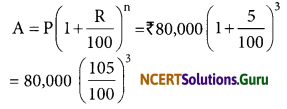= $$\frac{80000 \times 105 \times 105 \times 105}{100 \times 100 \times 100}$$
= ₹ 92610
∴ The required amount = ₹ 92610
Difference in amount = ₹ 92610 – ₹ 92400 = ₹ 210
Hence, difference in amount is ₹ 210.

Question 7.
Maria invested ₹ 8,000 in a business. She would be paid interest at 5% per annum compounded annually. Find
(i) The amount credited against her name at the end of the second year.
(ii) The interest for the 3rd year.
Solution:
(i) Here P = ₹ 8000, R = 5%, n = 2 yearsThe amount credited at the end of 2nd year = ₹ 8820

(ii) Here P = ₹ 8820, R = 5%, n = 1 year
Note: To find the interest for the 3rd year
(Find the S.I. for one year)
I = $$\frac{\text { PRT }}{100}$$
= $$\frac{8820 \times 5 \times 1}{100}$$
= ₹ 441
∴ Interest for the 3rd year is ₹ 441Question 8.
Find the amount and the compound interest on ₹ 10,000 for 1$$\frac{1}{2}$$ years at 10% per annum, compounded half-yearly. Would this interest be more than the interest he would get if it was compounded annually?
Solution:
If compounded half-yearly
Here P = ₹ 10,000
R = $$\frac{10}{2}$$ = 5% per half year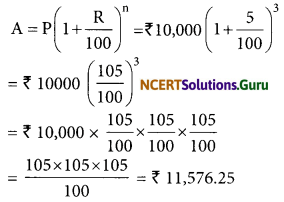C.I = A – P
= ₹ 11576.25 – ₹ 10,000
= ₹ 1576.25
₹ 1576.25 is the required C.I, when compounded half-yearly.

If compounded annually
Here, P = ₹ 10,000, R = 10%, n = 1 year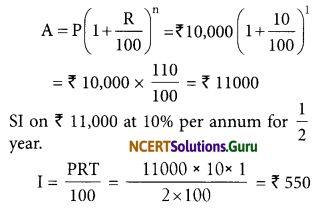Total compound Interest = ₹ (11000 – 10,000) + 550
= ₹ 1000 + ₹ 550
= ₹ 1550
When compounded half-yearly, the compound interest = ₹ 1576.25
When compounded annually, the compound interest = ₹ 1550
Hence, the interest when compounded half-yearly would be more than the interest when compounded annually.

Question 9.
Find the amount which Ram will get on ₹ 4096, if he gave it for 18 months at 12$$\frac{1}{2}$$% per annum, interest being compounded half-yearly.
Solution:Hence, the required amount ₹ 4913.Question 10.
The population of a place increased to 54,000 in 2003 at a rate of 5% per annum
(i) find the population in 2001.
(ii) what would be its population in 2005?
Solution:
Let the population in 2001 be ‘x’
R = 5%, n = 2years (2003 – 2001)
Population in 2003 is 54,000= 48979.59
= 48980 (approx).
Hence, the population in 2001 was about 48980

(ii) Here P = 54,000, R = 5% p.a., n = 2 years (2003 – 2005 = 2)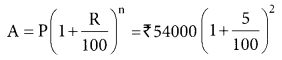Hence, the population in 2005 would be 59,535.

Question 11.
In a Laboratory, the count of bacteria in a certain experiment was increasing at the rate of 2.5% per hour. Find the bacteria at the end of 2 hours if the count was initially 5,06,000.
Solution:
Here P = 5,06,000, R = 2.5% per hour, n = 2 hours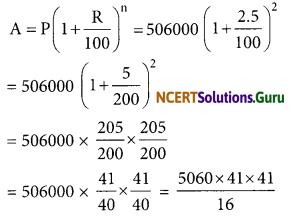= 531,616.25
= 531616 (approx).
Number of bacteria at the end of 2 hours = 531616 (approx.)Question 12.
A scooter was bought at ₹ 42,000. Its value depreciated at the rate of 8% per annum. Find its value after one year.
Solution:
Here P = ₹ 42,000 R = 8% p.a, n = 1 year
When the value depreciated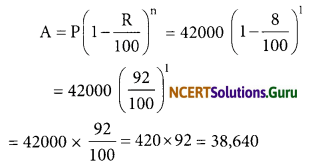Value of the scooter after one year = ₹ 38,640

error: Content is protected !!